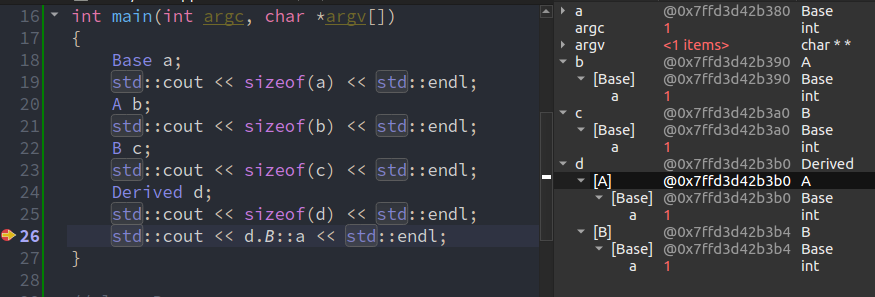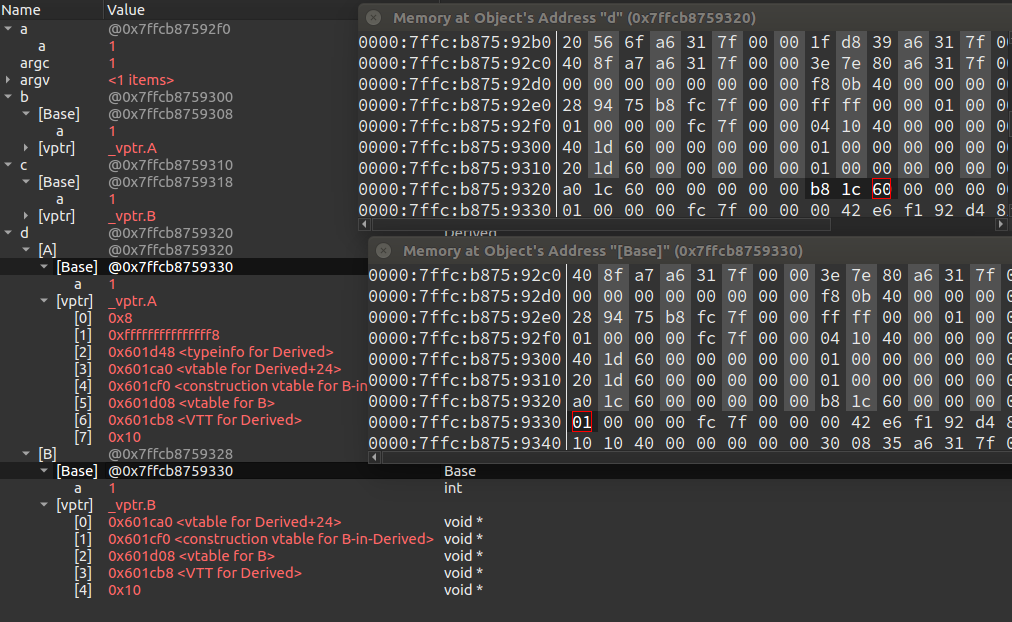# C++ 继承以及多继承

## 没啥事还是不要用多继承吧

Posted by kevin on October 24, 2019

## 继承

C++ 的继承有三种，分别是公有继承(public)，私有继承(private)，保护继承(protected)，一般情况下我们只会使用公有继承，几乎不会用到后面两种继承方式。

1. 基类的构造函数、析构函数和拷贝构造函数
2. 基类的重载运算符
3. 基类的友元函数

class derived-class: access-specifier base-class


## 多继承：

class Base
{
public:
void func()；
};

class A: public Base
{
public:
void func();
};

class B: public Base
{
public:
void func();
};

class Derived: public A, public B
{
public:
void func();
};


C++ 的多继承容易带来很多问题，因此在上课的时候老师也没怎么讲，自己之前理解也不到位，在这里看了很多资料之后整理了一下，将这些问题记录了下来。C++ 多继承最主要有两个问题，一个是同名二义性，另一个就是菱形继承带来的数据拷贝问题

### 菱形继承

class Base
{
public:
Base():a(1){}
int a;
};

class A: public Base{};

class B: public Base{};

class Derived: public A, public B{};

int main(int argc, char *argv[])
{
Base a;
std::cout << sizeof(a) << std::endl;
A b;
std::cout << sizeof(b) << std::endl;
B c;
std::cout << sizeof(c) << std::endl;
Derived d;
std::cout << sizeof(d) << std::endl;
}


int main(int argc, char *argv[])
{
Derived d;
std::cout << d.B::a << std::endl;
}


error: request for member ‘a’ is ambiguous
candidates are: int Base::a
note: int Base::a### 虚继承

class Base
{
public:
Base():a(1){}
int a;
};

class A: virtual public Base{};

class B: virtual public Base{};

class Derived: public A, public B{};

int main(int argc, char *argv[])
{
Base a;
std::cout << sizeof(a) << std::endl;
A b;
std::cout << sizeof(b) << std::endl;
B c;
std::cout << sizeof(c) << std::endl;
Derived d;
std::cout << sizeof(d) << std::endl;
}


### Attention

1) 结构体的首地址能够被其最宽基本类型成员的大小所整除

2) 结构体每个成员相对于结构体首地址的偏移量都是该成员大小的整数倍，如有需要，编译器会在成员之间加上填充字节；(当成员大小大于处理器位数时，偏移量应该为系统中最宽类型成员大小的整数倍)

3) 结构体的总大小为结构体最宽基本类型成员大小的整数倍，如有需要，编译器会在最末一个成员之后加上填充字节。(如果结构体最大成员的大小大于处理器位数，侧结构体的总大小为系统中最宽类型成员大小的整数倍)### 同名二义性

class Base
{
public:
Base():x(1){}
int x;
void print(){
std::cout << x << std::endl;
}
};

class A: virtual public Base{
public:
A():Base(),x(2){}
int x;
void print(){
std::cout << 233 << std::endl;
}
};

class B: virtual public Base{
public:
B():Base(),x(3){}
int x;
void print(){
std::cout << 666 << std::endl;
}
};

class Derived: public A, public B{};

int main(int argc, char *argv[])
{
Base a;
A b;
B c;
Derived d;
std::cout << d.x << std::endl;
d.print();
}



a.cpp:86:20: error: request for member ‘x’ is ambiguous
std::cout << d.x << std::endl;
^
a.cpp:72:9: note: candidates are: int B::x
int x;
^
a.cpp:63:9: note:                 int A::x
int x;
^
a.cpp:87:7: error: request for member ‘print’ is ambiguous
d.print();
^
a.cpp:73:10: note: candidates are: void B::print()
void print(){
^
a.cpp:64:10: note:                 void A::print()
void print(){
^



class Derived: public A, public B{
public:
void print(){
std::cout << "hhh" << std::endl;
}
};

int main(int argc, char *argv[])
{
Base a;
A b;
B c;
Derived d;
std::cout << d.B::x << std::endl;
d.B::print();
d.print();
}


## 总结

1. 虚继承解决的问题是菱形继承中多次基类内存拷贝的问题，并不能解决多重继承的二义性的问题，用虚继承防止了基类的数据在子类中存在多份拷贝的情况，也许上面的例子还不够清楚，因为上面的例子用了虚继承之后，对象占用的空间反而比不用虚继承还要大，这是因为我们上面只有一个数据成员，如果有很多数据成员的话，不用虚继承每次都要在子类中拷贝一份数据，浪费的空间可想而知，但是用了虚继承的话就只用在子类中添加一个虚基类表(一个指针)而已，可以大大减少空间的浪费，同时也解决了多重派生类调用基类数据产生的二义性(因为这时已经没有两个相同数据混淆编译器了)
2. 不要轻易相信网上的教程，很多都是假的，这种东西一定要自己尝试了调试过才知道背后的原理。不过没找到官方的菱形继承讲解，有的话最好还是看官方的
3. 一般不会用到多重继承，会用到的情况大概就是面试官考你的时候
4. 字节对齐要考虑到当前操作系统的位数
5. 同名二义性通过访问限定符和重写父类函数解决

## TODO

1. 搞懂为什么虚继承之后 d 的大小变成了 24 byte(因为涉及到字节对齐和操作系统位长)
2. 学会 GDB 之后再来调试一遍
3. 写一篇字节对齐的文章

## reference

https://www.jianshu.com/p/ab96f88e5285

https://blog.csdn.net/songshiMVP1/article/details/51173469

https://blog.csdn.net/liu_zhen_kai/article/details/81590467

https://blog.csdn.net/songshiMVP1/article/details/51173469

https://blog.csdn.net/chengonghao/article/details/51679743